# JavaScript Strings Tutorial with Examples

## 1. ECMAScript String

In ECMAScript, String is a special data type, because it is used frequently in a program, therefore, it is required to have performance and flexibility. That's reason why the String is both objective and primitive.
String Literal
You can create a string literal. The string literal is stored in a stack, requiring less storage space, and cheaper to manipulate.
``let aStringLiteral = "Hello World";``
string literals are contained in a Common Pool. When you declare two String Literal variables with the same content, they will point to the same address stored on the pool.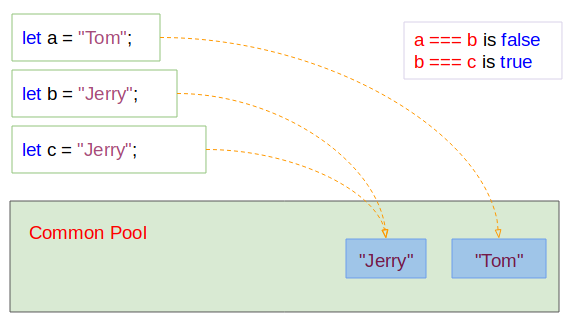string-literal-example.js
``````let a = "Tom";
let b = "Jerry";
let c = "Jerry";

console.log(a); // Tom
console.log(b); // Jerry
console.log(c); // Jerry

console.log( a === b); // false

console.log( b === c); // true``````
String Object
You can create a string through the constructor of the String class. In this case, you will receive a String object.
``let myStringObj = new String(value);``
Parameters:
• value: A value to convert to string
string-object-example.js
``````let a = new String("Hello World");
console.log(a); // [String: 'Hello World']

let b = new String(true);
console.log(b); // [String: 'true']

let c = new String(100.20);
console.log(c); // [String: '100.2']``````
The new operator always creates a new entity on the Heap memory, which explains why you have to spend more money using String Objects.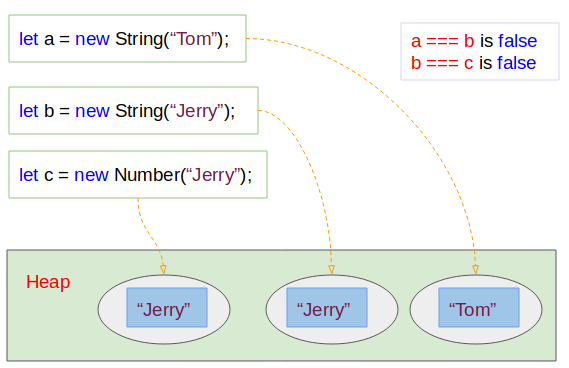When you assign a new value that is variable aa to variable bb. The variable bb will point to the address on memory to which the variable aa is pointing. There will not be an entity created on memory in this case.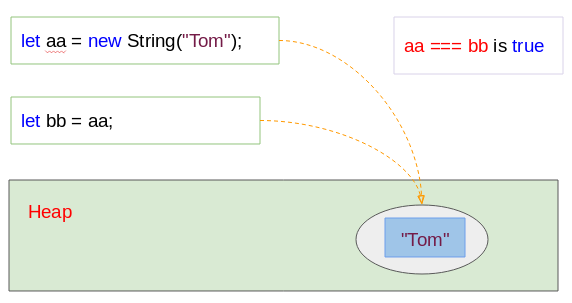string-object-example2.js
``````let aa = new String("Tom");

let bb = aa;

var sameAddress = aa === bb;

String object vs String Literal
String Literals are stored on Stack while String Objects are created and stored on the Heap memory. It requires complex memory management and storage space. So you should use String Literal instead of String Object at any possible time.
typeof
The typeof(string_object) operator will return 'object' string while typeof(string_literal) will return the 'string' string:
typeof-example.js
``````let myStringObj = new String("Tom");
console.log(myStringObj); // [String: 'Tom']
console.log( typeof(myStringObj) ); // object

let myStringLiteral =  "Jerry";
console.log(myStringLiteral); // Jerry
console.log( typeof(myStringLiteral) ); // string``````
Function - String(something)
The String(something) function convert something to String Literal.
String-function-example.js
``````// String Object:
let s = new String("Hello");
console.log(s); // [String: 'Hello']

// String(value) function:
// Convert String Object to String Literal.
let s2 = String(s);
console.log(s2); // Hello

// A Number Object.
let number = new Number(300.20);
let n2 = String(number);

console.log( n2 ); // 300.2``````

## 2. String is Imutable

In the ECMAScript, the String data type is imutable, which means that any action you do on the strings such as string concatenation, string cutting, etc. creates a new string on memory, or return another string.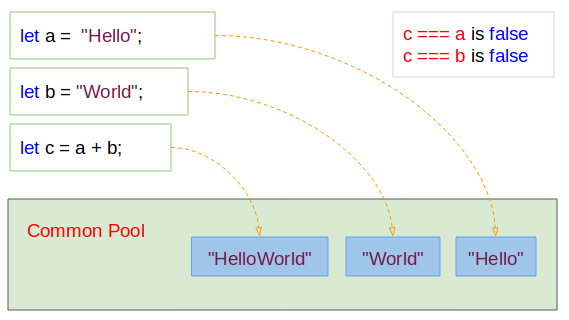The concat(string) method is used to concatenate a string into the current string. But in fact the current string does not change, and a new string (the result of concatenating) is created. The following figure illustrates program behavior: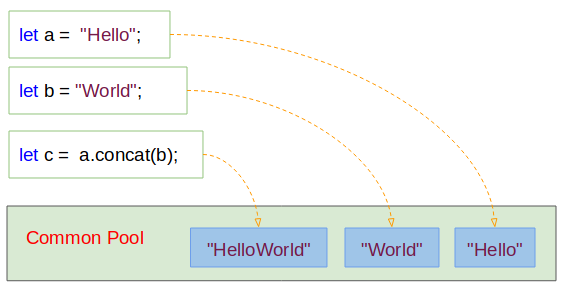concat-example2.js
``````let a = "Hello";
let b = "World";

let c = a.concat(b);

console.log(c); // HelloWorld
console.log(a); // Hello

console.log( a === c); // false``````

## 3. Properties of String

• constructor
• length
• prototype
length
This property returns the number of characters in a string.
length-example.js
``````let s1 = "Tom";

console.log(s1); // Tom
console.log("Length "+ s1.length); // 3

let s2 = "Tom & Jerry";

console.log(s2); // Tom & Jerry
console.log("Length "+ s2.length); // 11``````

## 4. Methods of String

charAt(index)
Returns the character at the specified index.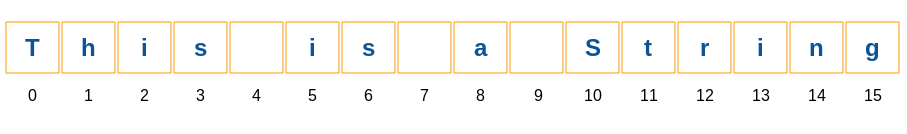charAt-example.js
``````let str = "This is a String";

console.log("str.charAt(0) is:" + str.charAt(0)); // T
console.log("str.charAt(1) is:" + str.charAt(1)); // h
console.log("str.charAt(2) is:" + str.charAt(2)); // i
console.log("str.charAt(3) is:" + str.charAt(3)); // s
console.log("str.charAt(4) is:" + str.charAt(4)); //
console.log("str.charAt(5) is:" + str.charAt(5)); // i``````
charCodeAt(index)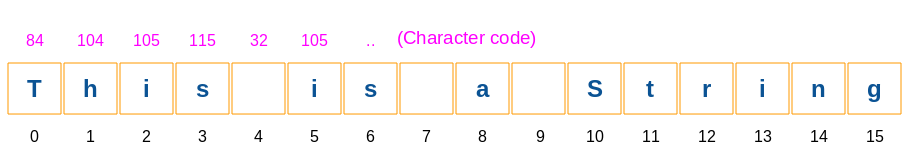charCodeAt-example.js
``````let str = "This is a String";

console.log("str.charCodeAt(0) is:" + str.charCodeAt(0)); // 84
console.log("str.charCodeAt(1) is:" + str.charCodeAt(1)); // 104
console.log("str.charCodeAt(2) is:" + str.charCodeAt(2)); // 105
console.log("str.charCodeAt(3) is:" + str.charCodeAt(3)); // 115
console.log("str.charCodeAt(4) is:" + str.charCodeAt(4)); // 32
console.log("str.charCodeAt(5) is:" + str.charCodeAt(5)); // 105``````
concat(string2[,string3[, ..., stringN]]
indexOf(searchValue[, fromIndex])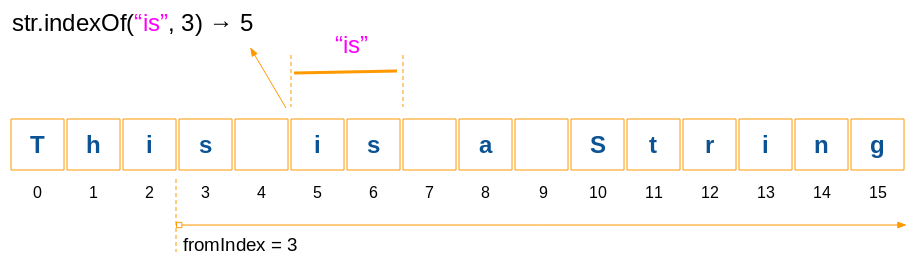indexOf-example.js
``````let str = "This is a String";

let idx = str.indexOf("is", 3);
console.log( idx ); // 5

let idx2 = str.indexOf("is");
console.log( idx2 ); // 2``````
lastIndexOf(searchValue[, toIndex])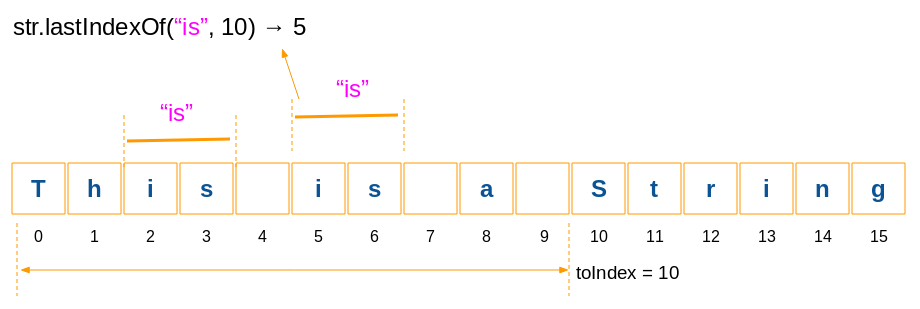lastIndexOf-example.js
``````let str = "This is a String";

let idx = str.lastIndexOf("is", 10);
console.log( idx ); // 5

let idx2 = str.lastIndexOf("is", 5);
console.log( idx2 ); // 5

let idx3 = str.lastIndexOf("is", 4);
console.log( idx3 ); // 4``````
str.localeCompare( otherStr )
This method is used to compare the content of current string and other string. Its purpose is to evaluate which string will precede, and which string succeeds. This method is useful when you want to arrange the order of strings. The comparison rules are based on the Locale of the environment.
returns:
• This method returns 0, which means that the 2 strings are the same .
• This method returns a number greater than 0 , which means that the current string is greater (succeeds) other string.
• This method returns a number less than 0, which means that the current string is less (precede) than other string.
localeCompare-example.js
``````//  "A" - "B" - "C"   ("A" < "B")
console.log( "A".localeCompare("B") ); // -1

// "B" > "A"
console.log( "B".localeCompare("A") ); // 1

// "Abc" < "bced"
console.log( "Abc".localeCompare("bced") ); // -1``````
str.replace(regexp/substr, newSubStr/function[, flags]);
Parameters:
• regexp − A RegExp object (Regular Expression). Substring matching the regular expression will be replaced by the return value of second parameter.
• substr − A String that is to be replaced by newSubStr.
• newSubStr − The String that replaces the substring received from first parameter.
• function − A function to be invoked to create the new substring.
• flags − A string containing attributes used for regular expressions (First parameter).
Example:
replace-example.js
``````let str = "Years: 2001, 2012, 2018";

// Replace first
let newStr = str.replace("20", "21");

console.log( newStr ); // Years: 2101, 2012, 2018``````
Example: Seek substrings that matches a regular expression and replace them with another substring.
replace-example2.js
``````let str = "Mr Blue has a blue house and a blue car";

// Replace (g: Global/All)
var res = str.replace(/blue/g, "red");

console.log(res);// Mr Blue has a red house and a red car``````
Example: Seek substrings that matches a regular expression and replace them with the value returned from a function.
replace-example3.js
``````var str = "Mr Blue has a blue house and a blue car";

function myfunc(subStr)  {
return subStr.toUpperCase();
}

// Replace (g: Global/All, i: Ignore Case)
var res = str.replace(/blue|house|car/gi,  myfunc);

console.log(res); // Mr BLUE has a BLUE HOUSE and a BLUE CAR``````
str.search(searchValue)
Seek substring positions matching a regular expression. If a value is not found -1 will be returned.
Parameter:
• searchValue - a regular expression or a string. If it is a string, it will automatically be converted into a regular expression.
search-example.js
``````// g: Global/All, i: Ignore Case
var regex = /apples/gi;

var str = "Apples are round, and apples are juicy.";

let idx = str.search(regex);

console.log(idx); // 0``````
str.slice( beginIndex [, endIndex] )
The method returns a substring determined by the two positions such as beginIndex and endIndex. If the beginIndex position is located on the right hand side and the endIndex is located on the left hand side, the returned string is always empty.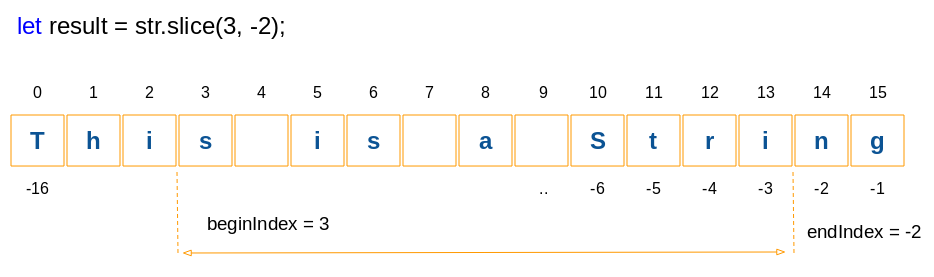slice-example.js
``````var str = "This is a String";

var result = str.slice(3, -2);

console.log(result);// s is a Stri

var result2 = str.slice(3);

console.log(result2);// s is a String

// IMPORTANT!!
var result3 = str.slice(5, 1); // ==> Empty String
console.log(result3);//

// IMPORTANT!!
var result4 = str.slice(5, -13); // ==> Empty String
console.log(result4);//``````
str.substr(start[, length])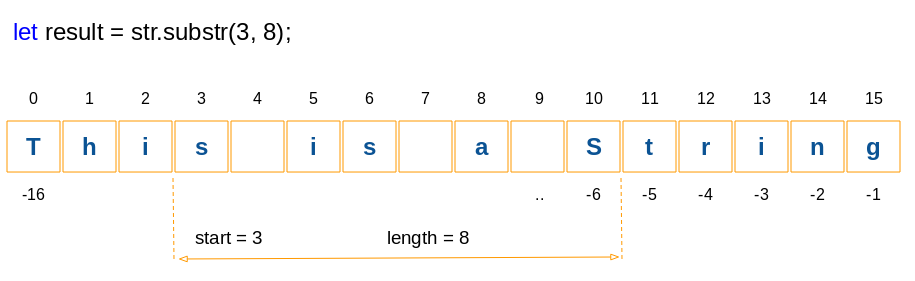substr-example.js
``````var str = "This is a String";

var result = str.substr(3, 8);

console.log(result);// s is a S

var result2 = str.substr(3);

console.log(result2);// s is a String``````
str.substring(beginIndex[, endIndex])
The method returns a substring defined by the two positions such as beginIndex and endIndex.. Note: substring(a, b) & substring(b, a) will return the same result.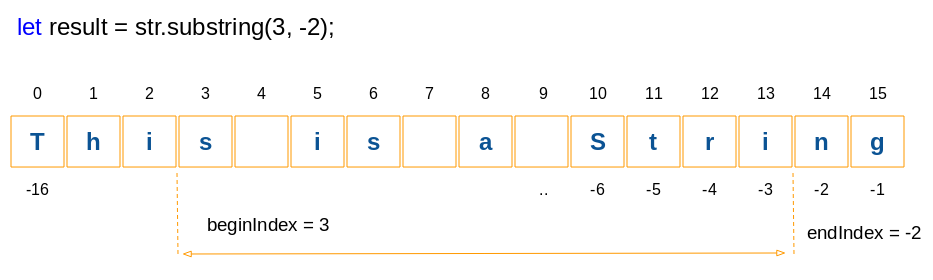substring-example.js
``````var str = "This is a String";

var result = str.substring(3, -2);

console.log(result);// s is a Stri

var result2 = str.substring(3);

console.log(result2);// s is a String

// IMPORTANT:
// result3 same as result4:
var result3 = str.substring(4, 1);
var result4 = str.substring(1, 4);

console.log(result3);// his
console.log(result4);// his``````
toLocaleLowerCase()
toLocaleLowerCase-example.js
``````let str = "This is a String";

var result = str.toLocaleLowerCase( );

console.log( result ); // this is a string``````
toLocaleupperCase()
toLocaleUpperCase-example.js
``````let str = "This is a String";

var result = str.toLocaleUpperCase( );

console.log( result ); // THIS IS A STRING``````
toLowerCase()
``````let str = "This is a String";

var result = str.toLowerCase( );

console.log( result ); // this is a string``````
toUpperCase()
toUpperCase-example.js
``````let str = "This is a String";

var result = str.toUpperCase( );

console.log( result ); // THIS IS A STRING``````
valueOf()
This method returns the String literal of a String object.
valueOf-example.js
``````let str = new String("This is a String");

console.log( str );// [String: 'This is a String']

let literal = str.valueOf();

console.log( literal );// This is a String``````